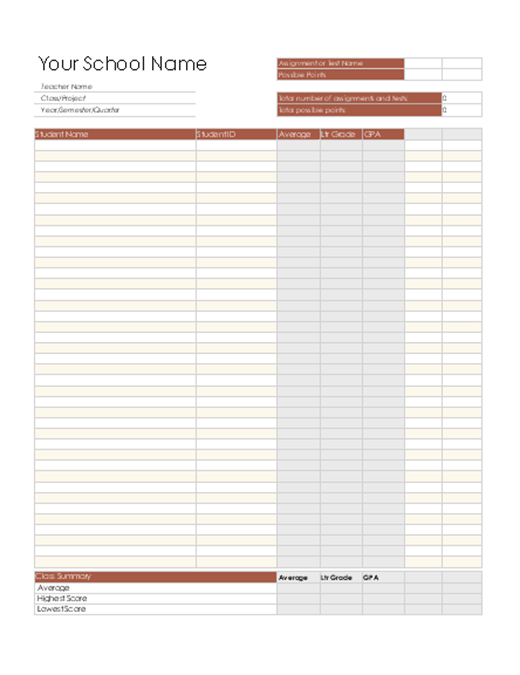Teacher's grade book based on points

Use this grade book template to determine grades where each assignment is worth a set of points. Averages, letter grades, and GPAs are calculated automatically.

Excel